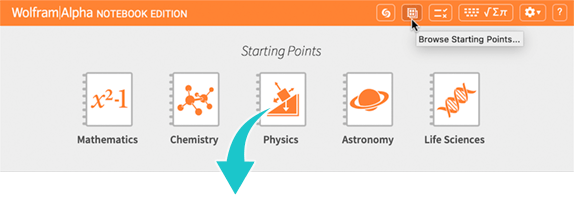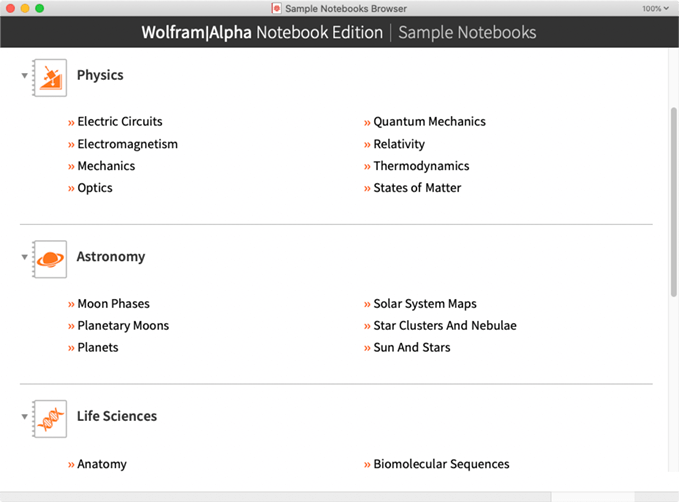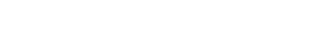The simplicity of Wolfram|Alpha with the computational capabilities of Mathematica.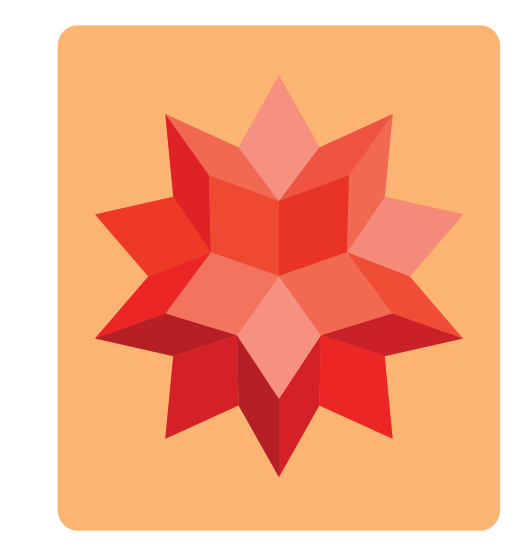New in Wolfram|Alpha Notebook Edition

2D typesetting for math constructs extends and simplifies query inputs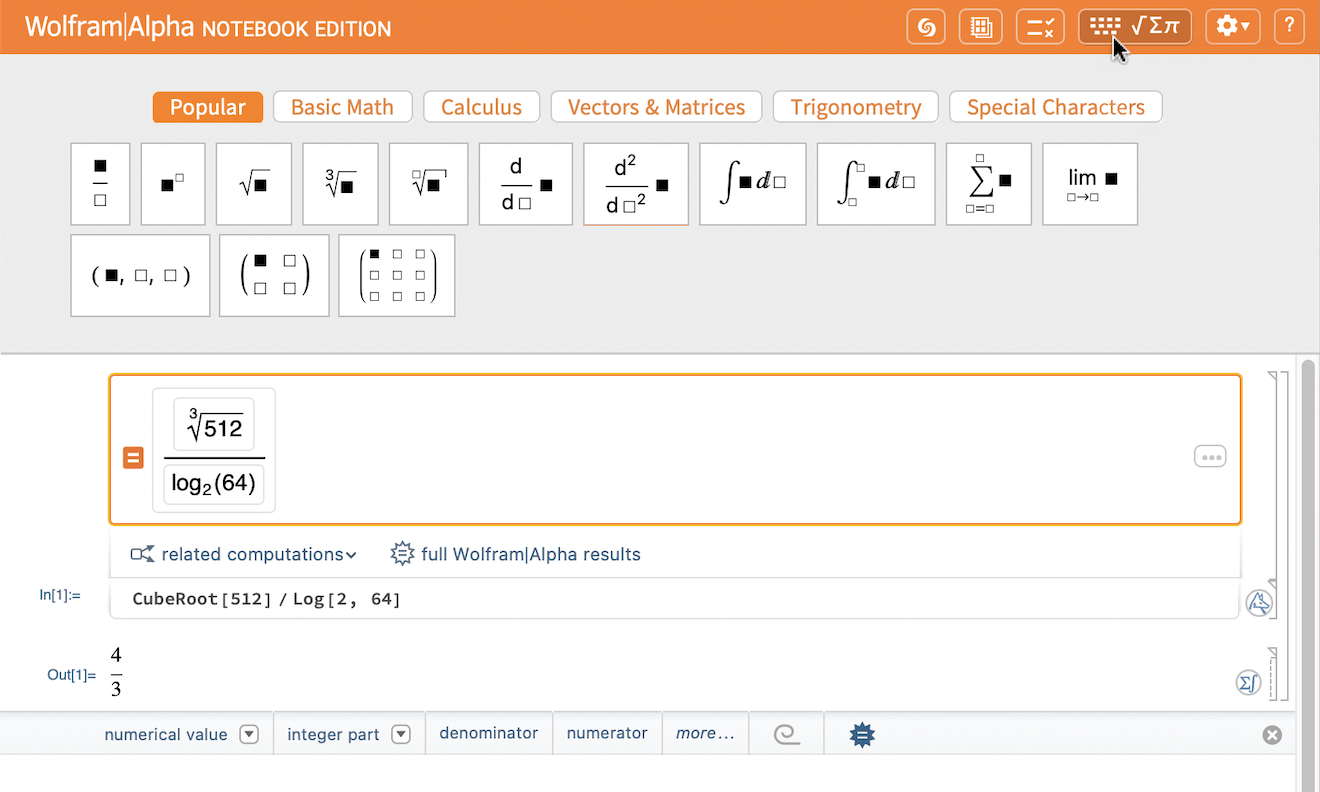Directly access and compute with biomolecular sequences via newly added natural language support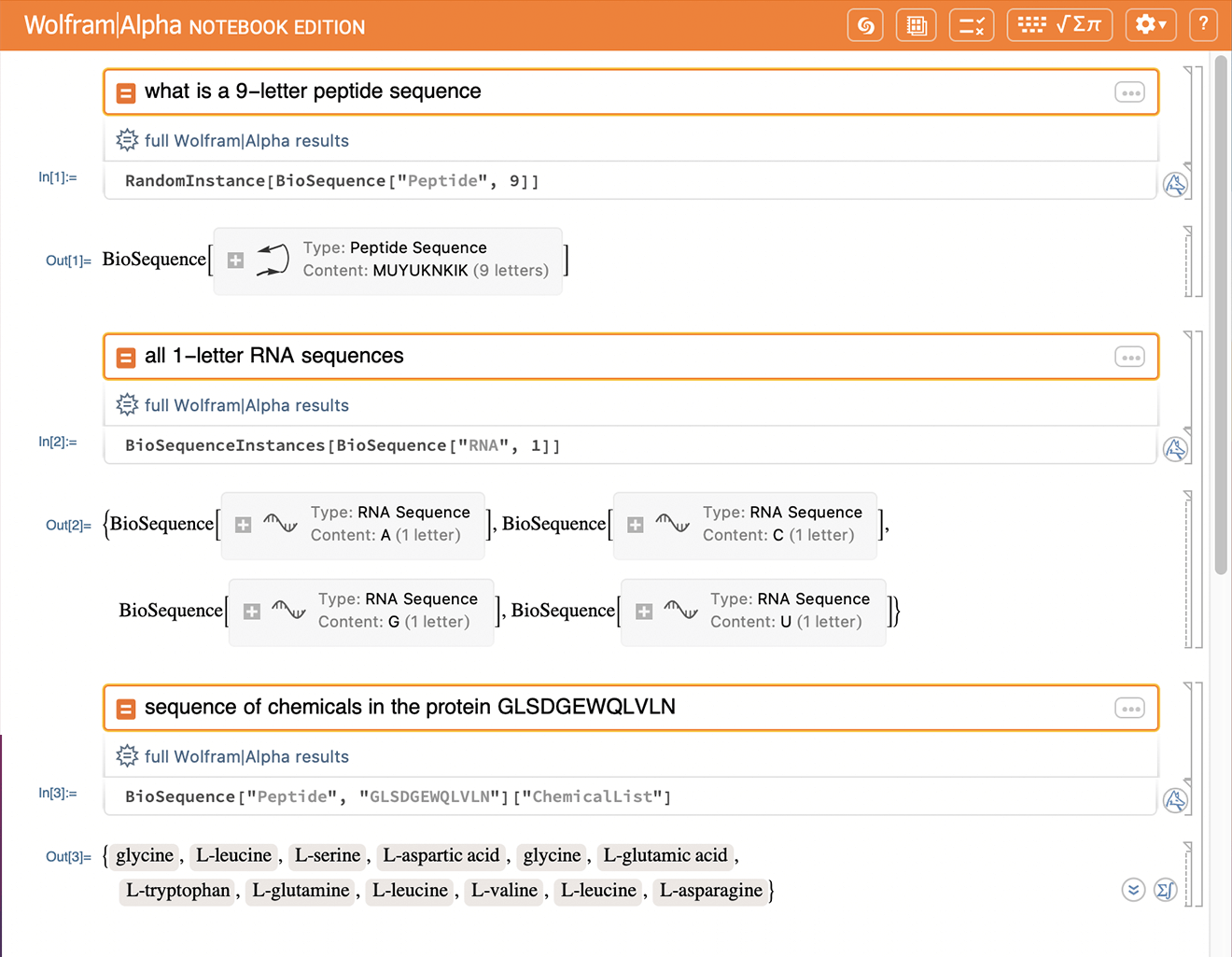Added and extended support for chemical computations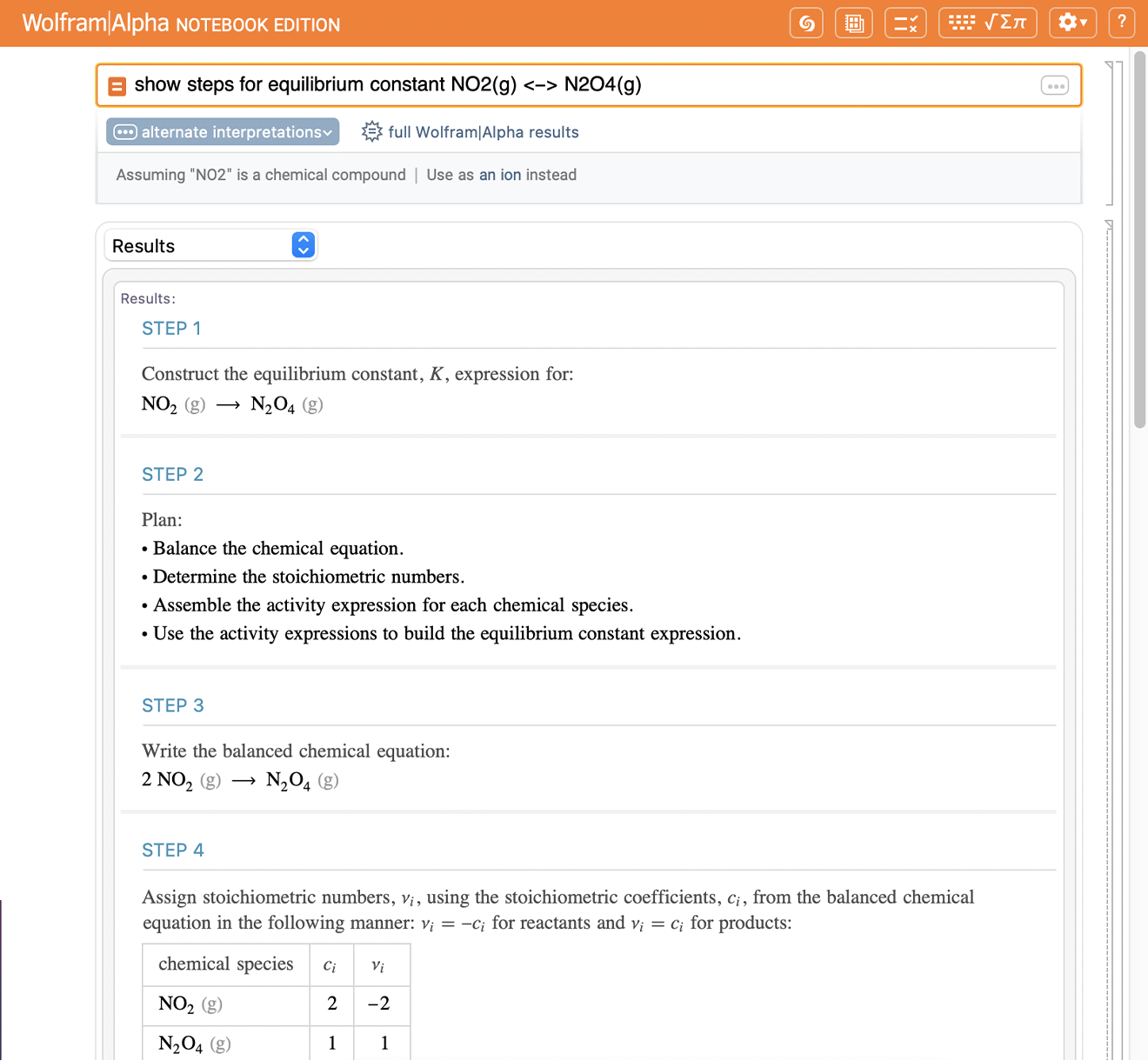Directly browse 12,000+ interactive Demonstration notebooks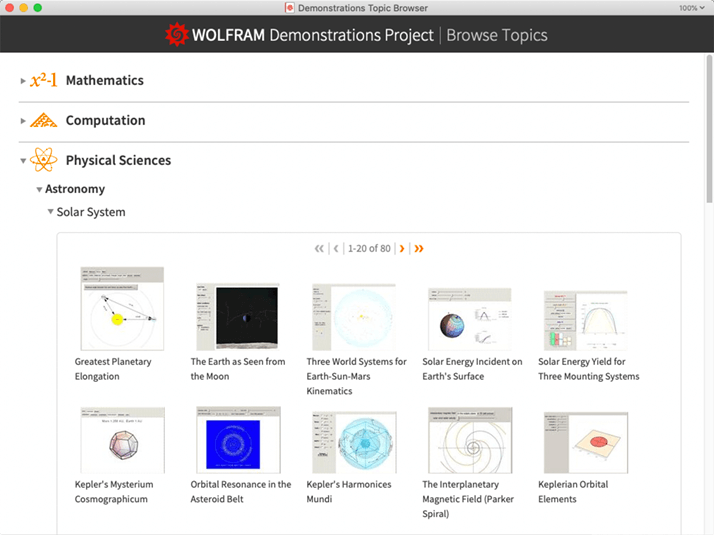Use the interactive plot quizzes to test your knowledge of mathematical functions and calculus concepts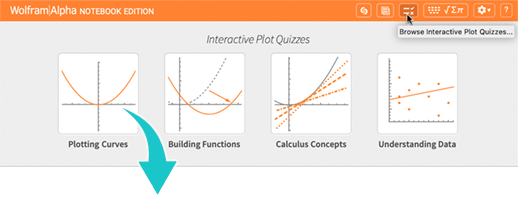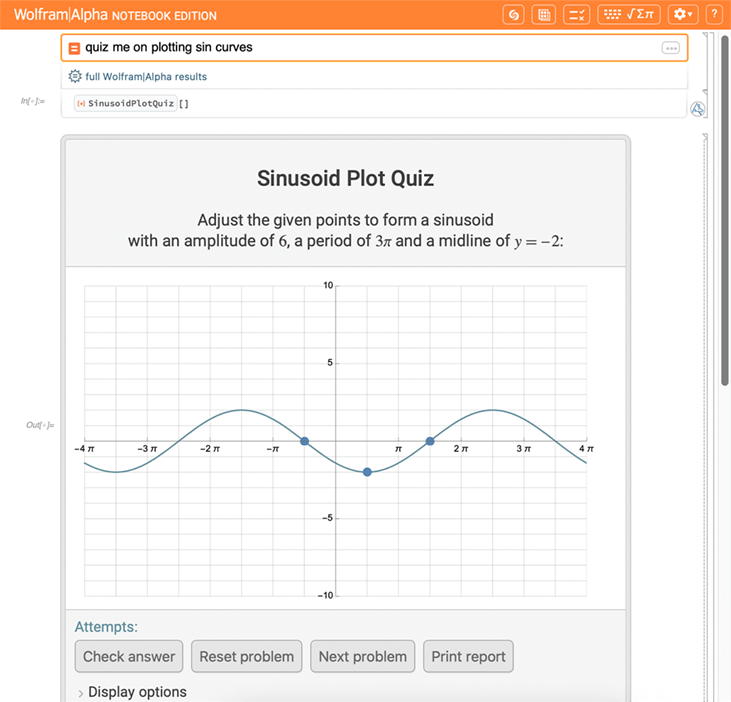Explore this collection of Wolfram|Alpha Notebook Edition notebooks to help you get started in different content areas